Author: Oscar Cronquist Article last updated on February 25, 2019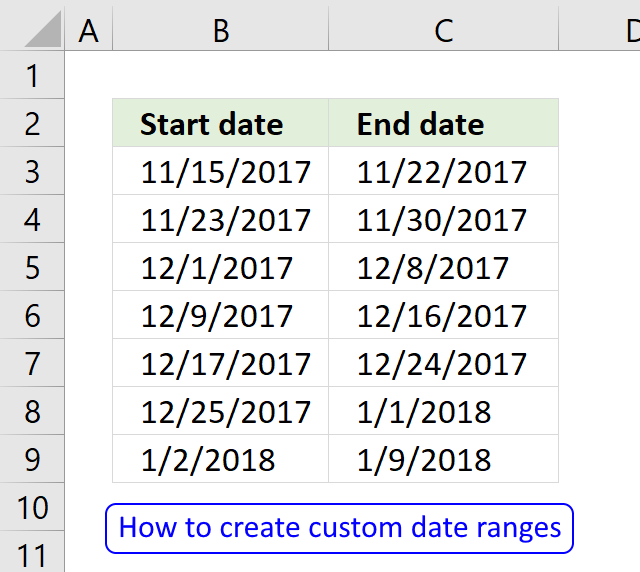Question: I am trying to create an excel spreadsheet that has a date range. Example:
Cell A1 1/4/2009-1/10/2009
Cell B1 1/11/2009-1/17/2009
Cell C1 1/18/2009-1/24/2009
How do I create a formula to do this?

I will in this article discuss what Excel dates actually are, how to use them in formulas and how to create a sequence of dates that you can use as date ranges.

### What are dates in Excel?

First, what are dates in Excel? They are actually numbers and I will prove it to you, try these steps: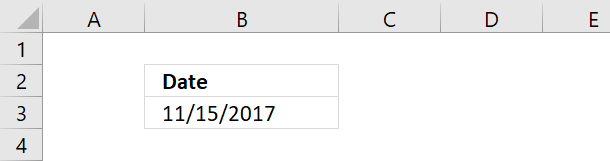1. Type a date in a cell
2. Select the cell
3. Press CTRL + 1 to open the "Format Cells" dialog box
4. Select "General"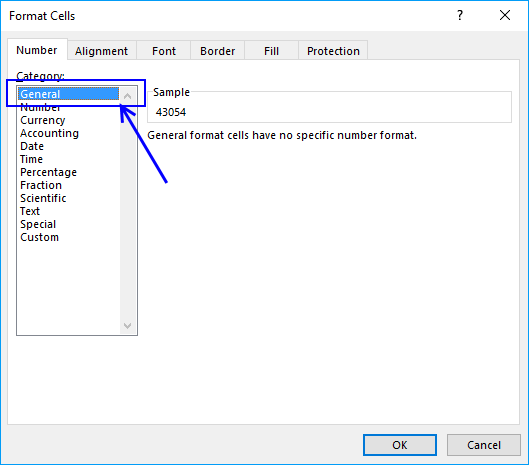5. Press with left mouse button on OK button
6. The cell you selected now has a different formatting. This shows you what dates are in Excel.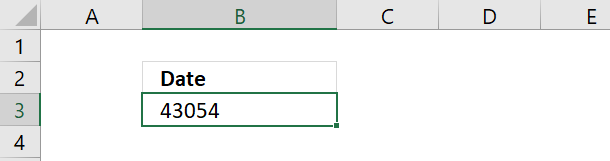Date 11/15/2017 is 43054. Excel starts numbering dates at 1/1/1900 with value 1. Type 1 in a cell and change the cell formatting to "Date" and see what Excel displays.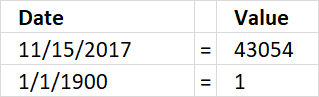Date 11/15/2017 is 43053 days from 1/1/1900. This means also that you can't use dates prior to 1/1/1900.

### Basic date ranges

You can build a formula or use a built-in feature to build date ranges, read on to learn more.

Now you know that dates in Excel are numbers. You can easily create a date range by adding a number to a date.

The picture below shows a start date 11/15/2017, adding number 7 to that date returns 11/22/2017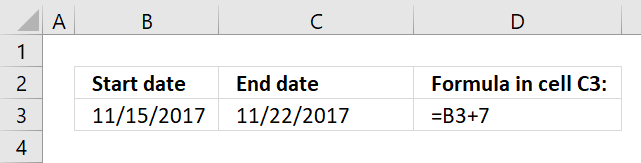This allows you to quickly build date ranges simply by adding a number to a date.

Now select cell B4 and type =C3+1

Copy cell C3 and paste to cell C4.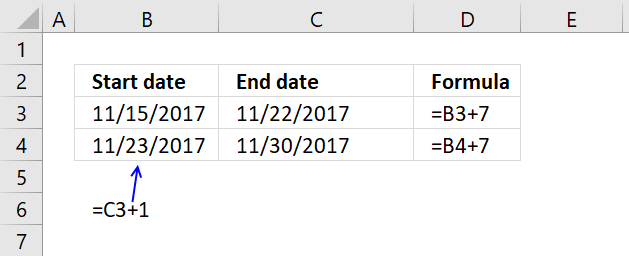Relative cell references changes when you copy a cell and paste it to a new cell and I am going to use that now.

Copy cell range B4:C4 and paste it to cells below.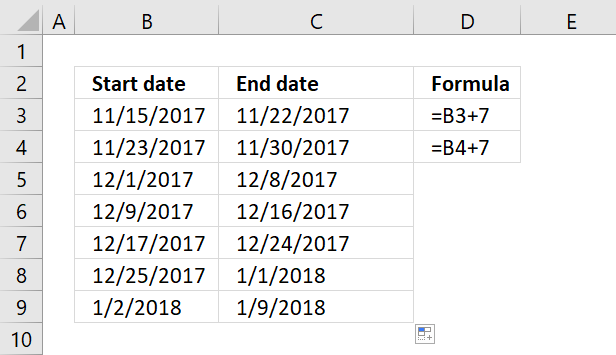You have now built multiple date ranges using simple mathematics.

#### Create a date sequence

Excel has a great built-in feature that allows you to create number sequences in no time. Since dates are numbers in Excel you can use the same technique to build date ranges.

To build date ranges that have the same range but dates change, follow these steps:

1. Type the start date and the end date in a cell each
2. Type the second start date an end date in cells below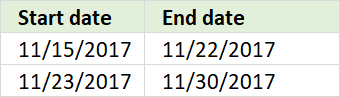3. Select both date ranges
4. Press and hold on black dot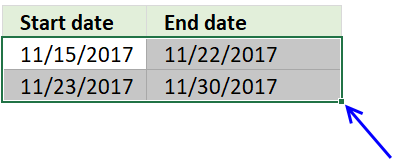5. Drag to cells below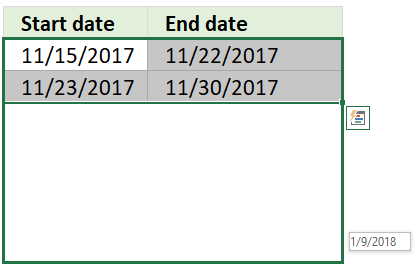6. Release mouse button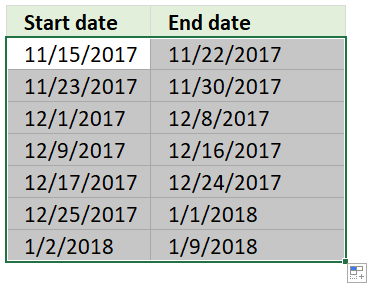Excel has now built date ranges automatically using the two first date ranges as a template.

#### Recommended articlesIf you need to build date ranges that have both the start and end date in the same cell you need to build a more complicated formula. The TEXT function lets you format dates out of numbers.

See row 3 in the above picture.

#### Date ranges horizontally

The following formula returns date ranges that exists after the start date, the start date in this case is 1/4/2009, however you can easily change that.

The DATE function creates the number using three arguments. The first argument is the year, the second argument is the month and the third argument is the day.

DATE(year, month, day)

Formula in cell A3:

=TEXT(DATE(2009, 1, 4)+(COLUMNS(\$A:A)-1)*7, "mm/dd/yy")&"-"&TEXT(DATE(2009, 1, 4)+(COLUMNS(\$A:A)-1)*7+6, "mm/dd/yy")

Copy cell A3 and paste to cells to the right as far as needed.

This formula returns date ranges that are prior to the given start date.

Formula in A6:

=TEXT(DATE(2009,1,4)+(2-COLUMN(A:A)-1)*7,"mm/dd/yy")&"-"&TEXT(DATE(2009,1,4)+(2-COLUMN(A:A)-1)*7+6,"mm/dd/yy")

#### Date ranges vertically

Formula in A9:

=TEXT(DATE(2009, 1, 4)+(ROW(1:1)-1)*7, "mm/dd/yy")&"-"&TEXT(DATE(2009, 1, 4)+(ROW(1:1)-1)*7+6, "mm/dd/yy")

Formula in A18:

=TEXT(DATE(2009, 1, 4)+(2-ROW(1:1)-1)*7, "mm/dd/yy")&"-"&TEXT(DATE(2009, 1, 4)+(2-ROW(1:1)-1)*7+6, "mm/dd/yy")

#### How the formula in cell A3 works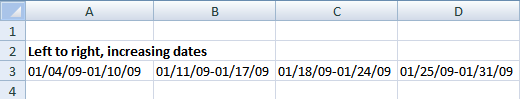The goal with the formula above is to create a date range from Sunday to Saturday. When cell A3 is copied and pasted to the right, the date range adjusts accordingly.

The formula consists of two parts. The first part calculates the start date of the date range. I have bolded the start date part of the formula below.

=TEXT(DATE(2009, 1, 4)+(COLUMNS(\$A:A)-1)*7, "mm/dd/yy")&"-"&TEXT(DATE(2009, 1, 4)+(COLUMNS(\$A:A)-1)*7+6, "mm/dd/yy")

#### Step 1 - First part of the formula creates the start date (bolded)

I have bolded the start date in this date range: 01/04/09 - 01/10/09.

TEXT(DATE(2009, 1, 4)+(COLUMNS(\$A:A)-1)*7, "mm/dd/yy")

DATE(2009, 1, 4) is the start date of the date range series.

DATE(2009, 1, 4) returns 39817

(COLUMN(A:A)-1)*7 creates a number determined by the current column.

COLUMNS(\$A:A) returns 1.

COLUMNS(\$A:A) - 1 returns 0.

(COLUMNS(\$A:A)-1)*7 returns 0.

This number creates how many days there is between start dates in the date ranges.

Start dates are bolded below.

Example

01/04/09 - 01/10/09 ; 01/11/09 - 01/17/09 ; 01/18/09 - 01/10/09

11 - 4 = 7, 18 - 11 = 7.

When the formula is copied and pasted to the right, the relative reference in COLUMNS(\$A:A) changes.

This cell reference changes as the formula is copied and pasted to the right.

Example,

COLUMNS(\$A:A) is 1

COLUMNS(\$A:B) is 2

COLUMNS(\$A:C) is 3

TEXT(DATE(2009, 1, 4)+0, "mm/dd/yy")

becomes

TEXT(39817 + 0, "mm/dd/yy").

"M/DD/YY" is the specified number format.

TEXT(39817 + 0, "mm/dd/yy") returns 01/04/09

#### Step 2 - Second part of the formula creates the end date

The second part of the formula is

TEXT(DATE(2009, 1, 4)+(COLUMNS(\$A:A)-1)*7+6, "mm/dd/yy")

The only difference between the first part of the formula:

TEXT(DATE(2009, 1, 4)+(COLUMNS(\$A:A)-1)*7, "mm/dd/yy")

and the second part of the formula:

TEXT(DATE(2009, 1, 4)+(COLUMNS(\$A:A)-1)*7+6, "mm/dd/yy")

is +6 (bolded)

Example

01/04/09 - 01/10/09

10 - 4 = 6